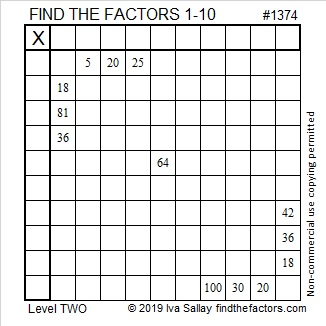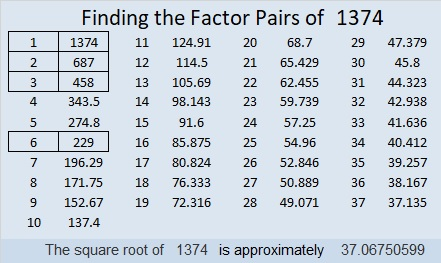# 1374 and Level 2

Can you find the factors that will turn this puzzle into a multiplication table whose products are simply not in the usual order?Print the puzzles or type the solution in this excel file: 10 Factors 1373-1388

Here are a few facts about the puzzle number, 1374:

• 1374 is a composite number.
• Prime factorization: 1374 = 2 × 3 × 229
• 1374 has no exponents greater than 1 in its prime factorization, so √1374 cannot be simplified.
• The exponents in the prime factorization are 1, 1, and 1. Adding one to each exponent and multiplying we get (1 + 1)(1 + 1)(1 + 1) = 2 × 2 × 2 = 8. Therefore 1374 has exactly 8 factors.
• The factors of 1374 are outlined with their factor pair partners in the graphic below.1374 is the hypotenuse of a Pythagorean triple:
360-1326-1374 which is 6 times (60-221-229)

This site uses Akismet to reduce spam. Learn how your comment data is processed.